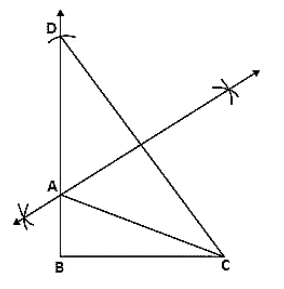# Construct a right-angled triangle ABC whose base BC is 6 cm and`
Question:

Construct a right-angled triangle ABC whose base BC is 6 cm and the sum of the hypotenuse AC and other side AB is 10 cm.

Solution:

Steps of Construction:

1. Construct a line segment BC of 6 cm.

2. At the point B, draw ∠XBC = 90°.

3. Keeping B as center and radius 10cm draw an arc which intersects XB at D.

4. Join DC.

5. Draw the perpendicular bisector of DC which intersects DB at A.

6. Join AC.

Hence △ABC is the required triangle.